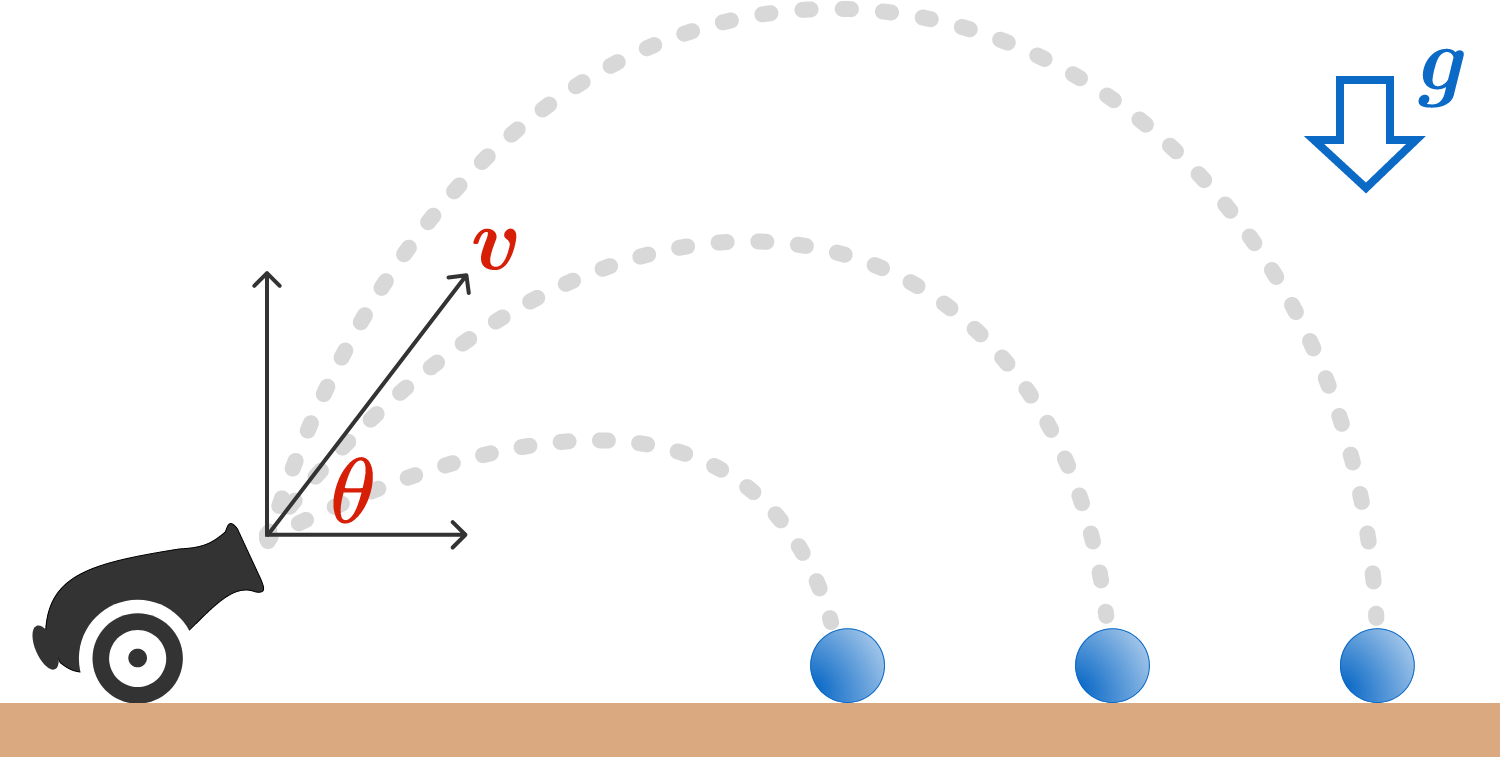# Average Landing Distance (Part 2)

A massive particle is launched from ground level with a velocity of magnitude $v$ and a launch angle of $\theta$ with respect to the ground.

Suppose a large (essentially infinite) number of launches take place. Over the many trials, $\theta$ varies uniformly between $0$ and $\frac{\pi}{2}$, and $v$ varies uniformly between 0 and $v_\text{max}$.

If there is a uniform downward gravitational acceleration $g$, the expected average distance of the landing point from the launch point (assuming level ground) can be expressed as $\dfrac{a}{b} \dfrac{v_\text{max}^{2}}{\pi g}$, where $a$ and $b$ are coprime positive integers.

Determine $a+b$.×